In :
# %load ../../preconfig.py
%matplotlib inline
import matplotlib.pyplot as plt
import seaborn as sns
plt.rcParams['axes.grid'] = False

from __future__ import division

#import numpy as np
#import pandas as pd
#import itertools

import logging
logger = logging.getLogger()


# 18 B-Trees¶

B-Trees are balanced search trees, similar to red-black trees, but they are better at minizing disk I/O operations.

We use the number of pages read or written as a approximation of the total time spent accessing the disk.

A B-tree node is usually as large as a whole disk page, and this size limits the number of children a B-tree node can have.

In :
plt.imshow(plt.imread('./res/fig18_3.png'))

Out:
<matplotlib.image.AxesImage at 0x109444c50>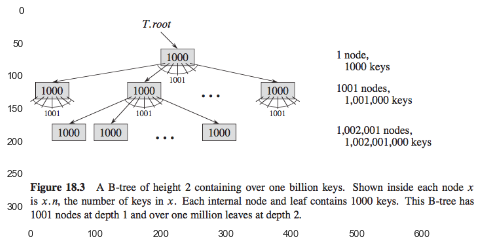### 18.1 Definition of B-trees¶

#### Properties¶

1. node attributes:

• $x.n$: the number of keys.
• $x.\text{keys}$: $x.\text{key}_1 \leq x.\text{key}_2 \leq \dotsb \leq x.\text{key}_n$.
• $x.leaf$: True if it is, False otherwise.
2. Each internal node has $x.n+1$ points $x.c_1, \dotsc, x.c_{x.n+1}$ to its children.

3. $x.c_i.keys \leq x.key_i \leq x.c_{i+1}.keys$

4. All leaves have the same depth $h$.

5. minimum degree of the B-tree $T$: a fixed integer $t \geq 2$.

• Every node other than the root must have at least $t-1$ keys.
• Every node may contain at most $2t-1$ keys. FULL.

The simplest B-tree occurs when $t=2$: 2-3-4 tree.

#### The worst-case height of B-tree¶

If $n \geq 1$, then for any $n$-key B-tree $T$ of height $h$ and minimum degree $t \geq 2$.
$$h \leq \log_t \frac{n+1}{2}$$

Proof: The root of a B-tree $T$ contains at least one key, and all other nodes contain at least $t-1$ keys.

In :
plt.figure(figsize=(15,10))

Out:
<matplotlib.image.AxesImage at 0x10a750fd0>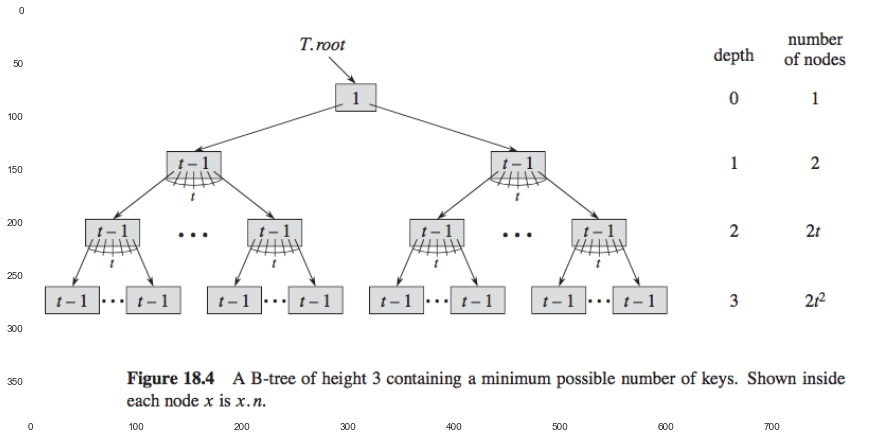the number $n$ of keys statisfies the inequality: $$n \geq 1 + (t-1) \sum_{i=1}^h 2t^{i-1} = 2t^h -1$$

B-trees save a factor of about $\lg t$ over red-black trees in the number of nodes examined for most tree operations.

%maybe: exercise

### 18.2 Basic operations on B-trees¶

two conventions:

1. The root of the B-tree is always in main memory.

2. Any nodes that are passed as parameters must already have had a Disk-Read operation performed on them.

#### Searching a B-tree¶

We make a multiway branching decision according to the number of the node's children. $O(t lg_t n) = O(t) \times O(h)$.

In :
#todo: code


#### Creating an empty B-tree¶

$O(1)$ time to allcate one disk page.

In :
#todo: code


#### Inserting a key into a B-tree¶

As we trave down the tree, we split each full node we meet, in order to assure that the parent is always not full whenever we want to split a full node.

(a) B-TREE-SPLIT-CHILD(X,I)

In :
plt.imshow(plt.imread('./res/fig18_5.png'))

Out:
<matplotlib.image.AxesImage at 0x10bfee110>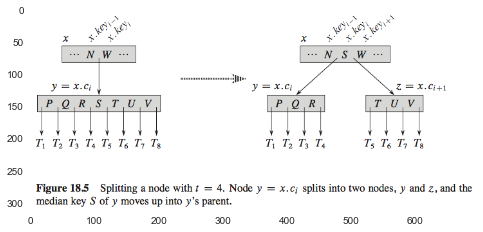If $T$ is full tree, then create a new root node, and split them. (Height increase 1).

(b)

B-TREE-INSERT(T,K)
if T is FULL:
S = B-TREE-CREATE()
S.children = T
R = B-TREE-SPLIT-CHILD(S)
else
R = T

B-TREE-INSERT-NONFULL(R,K)
In :
plt.imshow(plt.imread('./res/fig18_6.png'))

Out:
<matplotlib.image.AxesImage at 0x10c1cfe90>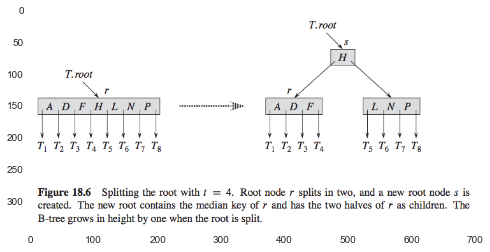(c)

B-TREE-INSERT-NONFULL(T,K)
if T is LEAF
FIND_AND_INSERT(T,K)
else
C = FIND_CHILD(T,K)
if C is FULL
N = B-TREE-SPLIT-CHILD(C)
R = FIND_CHILD(N,K)
else
R = T

B-TREE-INSERT-NONFULL(R, K)
In :
#todo: exercises


### 18.3 Deleting a key from a B-tree¶

1. When going down, if the node's child has only $t-1$ keys, move its key down to the child.

• if the node is root, then delete the root, use its child as new root. (Height -1).
2. If the node to delete is a leaf node, then directly remove it.

3. If the node to delete is a internal node, then

• If the key's left child has more than $t-1$ keys, move up the lastest key.
• If the key's right child has more than $t-1$ keys, move up the first key.
• otherwise, the sum of left and right child's keys is less than $2t-1$, merge them as a new node.

Base on the three priciples, we summary all possible cases as follows:

In :
plt.figure(figsize=(10,20))
plt.subplot(2,1,1)

<matplotlib.image.AxesImage at 0x10ce6e610>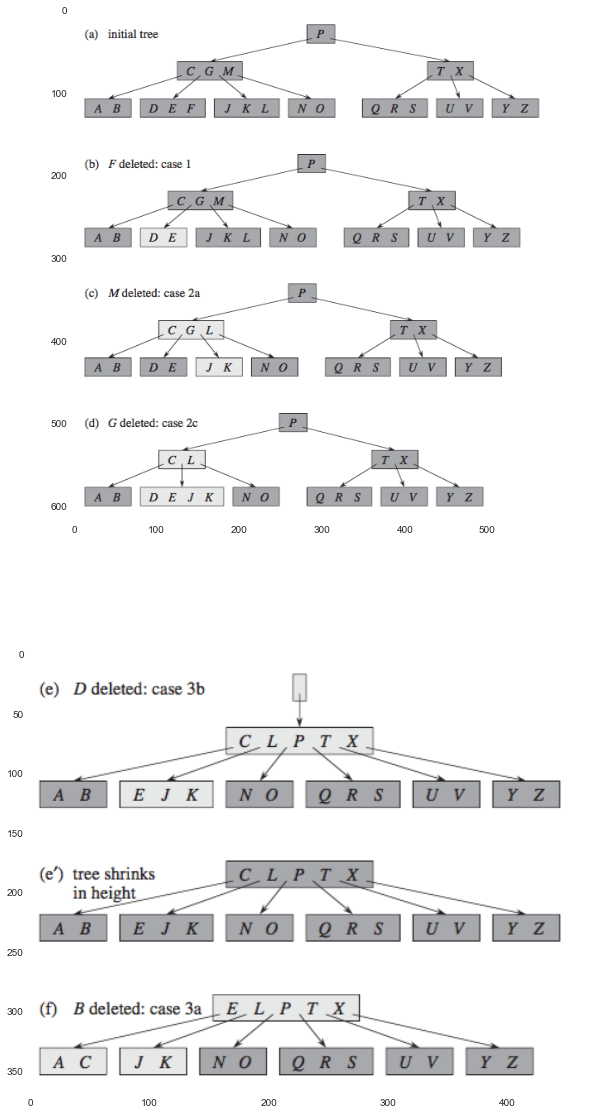#todo: exercise